# Precalculus : Algebra of Functions

## Example Questions

1 2 3 4 6 Next →

### Example Question #26 : Composition Of Functions

Suppose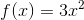and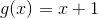.  Find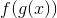.

Possible Answers: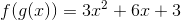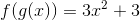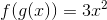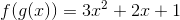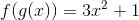Correct answer:Explanation:

To find, you must subsititue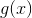into the function,.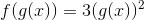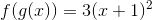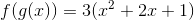### Example Question #27 : Composition Of Functions

If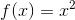and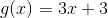, what is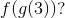Possible Answers:Correct answer:Explanation:

First, we find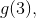or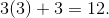Then, find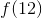, or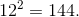### Example Question #28 : Composition Of Functions

Given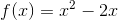and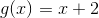find.

Possible Answers: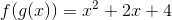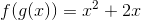None of these.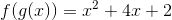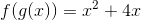Correct answer:Explanation:

Findingis the same as plugging inintomuch like one would find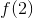for a function.andInsert g(x) into f(x) everywhere there is a variable in f(x):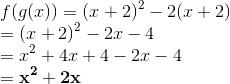### Example Question #29 : Composition Of Functions

We are given the following: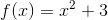and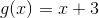.

Find:Possible Answers:

None of the other answers.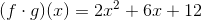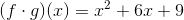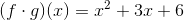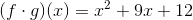Correct answer:

None of the other answers.

Explanation:

Let's discuss what the problem is asking us to solve. The expression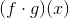(read as as "f of g of x") is the same as. In other words, we need to substituteintoSubstitute the equation offor the variable in the givenfunction:Next we need to FOIL the squared term and simplify: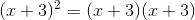FOIL means that we multiply terms in the following order: first, outer, inner, and last.

First: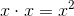Outer: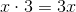Inner: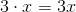Last: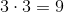When we combine like terms, we get the following: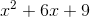Substitute this back into the equation and continue to simplify.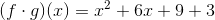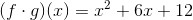None of the answers are correct.

1 2 3 4 6 Next →

### All Precalculus Resources